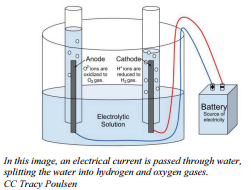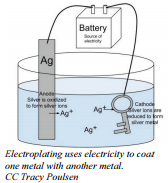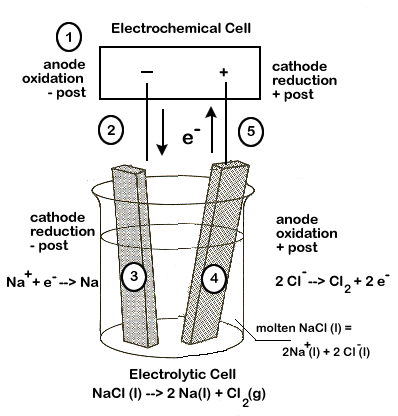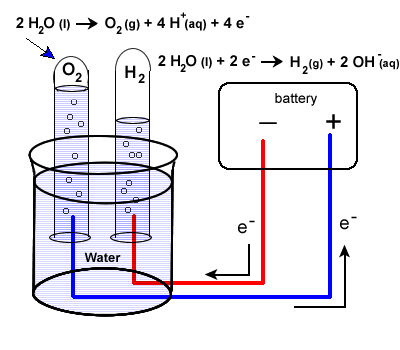# 16.7: Electrolysis: Using Electricity to Do Chemistry

Learning Objectives

• Explain the following laws within the Ideal Gas Law

So far we have discussed how electricity can be produced from chemical reactions in batteries. Some reactions will, instead, use electricity to get a reaction to occur. In these reactions, electrical energy is given to the reactants causing them to react to form the products. These reactions have many uses. For example, electrolysis is a process that involves forcing electricity through a liquid or solution to cause a reaction to occur. Electrolysis reactions will not run unless energy is put into the system from outside. In the case of electrolysis reactions, the energy is provided by the battery. Think of electrolysis and electrolytic cells as the opposite of electrochemical cells:

Electrochemical Cells
Electrolytic Cells
Energy conversion
Chemical → Electrical
Electrical → Chemical
Spontaneous chemical reaction?
Yes
No
Value of E°
Positive
Negative

In an electrochemical cell, a spontaneous redox reaction is used to create an electric current; in an electrolytic cell the reverse will occur - an electric current will be required in order to cause a non-spontaneous chemical reaction to occur. We will look at three examples of the electrolytic process, keeping our discussion on a very basic level - the electrolysis of molten sodium chloride, the electrolysis of water, and electroplating.Figure 16.7.1: An electrical current is passed through water, splitting the water into hydrogen and oxygen dases.

If electrodes connected to battery terminals are placed in liquid sodium chloride, the sodium ions will migrate toward the negative electrode and be reduced while the chloride ions migrate toward the positive electrode and are oxidized. The processes that occur at the electrodes can be represented by what are called half-equations.

Reduction occurs at the positive electrode:

$\ce{Na^+} + \ce{e^-} \rightarrow \ce{Na}$

Oxidation occurs at the negative electrode:

$2 \ce{Cl^-} \rightarrow \ce{Cl_2} + 2 \ce{e^-}$

The overall reaction for this reaction is:

$2 \ce{Na^+} + 2 \ce{Cl^-} \rightarrow 2 \ce{Na} + \ce{Cl_2}$

With appropriate treatment from the battery, it is possible to get the metal being reduced in an electrolysis process to adhere strongly to the electrode. The use of electrolysis to coat one material with a layer of metal is called electroplating. Usually, electroplating is used to cover a cheap metal with a layer of more expensive and more attractive metal. Many girls buy jewelry that is plated in gold. Sometimes, electroplating is used to get a surface metal that is a better conductor of electricity. When you wish to have the surface properties of gold (attractive, corrosion resistant, or good conductor) but you don't want to have the great cost of making the entire object out of solid gold, the answer may be to use cheap metal to make the object and then electroplate a thin layer of gold on the surface.Figure 16.7.2: Silver platting.

To silver plate an object like a spoon (silverware that's plated is less expensive than pure silver), the spoon is placed in the position of the cathode in an electrolysis set up with a solution of silver nitrate. When the current is turned on, the silver ions will migrate through the solution, touch the cathode (spoon) and adhere to it. With enough time and care, a layer of silver can be plated over the entire spoon. The anode for this operation would often be a large piece of silver from which silver ions would be oxidized and these ions would enter the solution. This is a way of ensuring a steady supply of silver ions for the plating process.

• Half-reaction at the cathode:

$\ce{Ag^+} + \ce{e^-} \rightarrow \ce{Ag}$

• Half-reaction at the anode:

$\ce{Ag} \rightarrow \ce{Ag^+} + \ce{e^-}$

Some percentage of the gold and silver jewelry sold is electroplated. The connection points in electric switches are often gold plated to improve electrical conductivity and most of the chromium pieces on automobiles are chromium plated.

## Electrolysis of Molten Sodium Chloride

If we look at the latin roots of the word "electrolysis" we learn that it means, essentially, to "break apart" (lysis) using electricity. Our first example of electrolytic cell will examine how an electric current can be used to break apart an ionic compound into its elements. The following equation represents the breaking apart of NaCl(l):

2NaCl(l) → 2Na(l) + Cl2 (g)

The half-reactions involved in this process are:

 E° reduction 2Na+(l) + 2e- → Na(s) -2.71 V oxidation Cl-(l) → Cl2 (g) + 2 e- -1.36V net voltage required - 4.07V

Notice that a negative voltage (-4.07V) results when we add up the half-reactions. This tells us that the overall reaction will NOT be spontaneous, and a minimum of 4.07 volts will be required for this reaction to occur.

As we shall see, our set-up will have a number of similarities to our electrochemical cells. We will need electrodes and an electrolyte to carry the electric current.

In our NaCl example, the electrodes will simply carry the current, but otherwise not be directly involved in the reaction. The electrolyte will be the actual molten (melted) NaCl. The electrodes and electrolyte are both required to carry the electric current. Molten NaCl must be used because solid ionic compounds do not carry an electric charge.

Some key differences with an electrochemical cell set-up:

• the two half-reactions are not separated by a salt bridge
• an electrochemical cell (or other source of electric current) will be required

Other important items to note:

• The anode of the electrolytic cell is the site of oxidation and the cathode is the site of reduction, just as in an electrochemical cell.
• In an electrochemical cell, the anode is negative and cathode positive, but this is reversed in the electrolytic cell - the anode is positive and the cathode is negative.

Carefully study the diagram of our set-up, taking special care to trace the path of the electrons. Unless electrons make a complete circuit, a reaction will not occur.

1. Electrons are "produced" in the battery at the anode, the site of oxidation.
2. The electrons leave the electrochemical cell through the external circuit.
3. These negative electrons create a negative electrode in the electrolytic cell which attracts the positive Na+ ions in the electrolyte. Na+ ions combine with the free electrons and become reduced (2Na+ + 2e- → Na )
4. Meanwhile the negative Cl- become attracted to the positive electrode of the electrolytic cell. At this electrode chlorine is oxidized, releasing electrons (Cl-→ Cl2 + 2 e-)
5. These electrons travel through the external circuit, returning to the electrochemical cell.## Electrolysis of Water

Our second example of electrolysis and electrolytic cells involves the breakdown of water. We will find a situation very similar to the electrolysis of molten NaCl. The following equation represents the breaking apart of H2O(l):

2H2O(l) → 2H2(g) + O2 (g)

It may be more difficult to predict the half-reactions involved, but they are:

 E° reduction 2H2O(l) + 2e- → H2 + 2 OH- -0.83 V oxidation 2H2O(l) → O2 + 4H+ + 4e- -1.23V (see note below for net equation) net voltage required - 2.06V

The set-up will be very similar to our last example with some minor differences. Water does not carry a charge well, so an electrolyte is added to the water. Vinegar, a weak acid (acetic acid) may be used. To collect the hydrogen and oxygen gases produced, inverted test tubes are often added, as shown in our diagram below.

Again, take special care to trace the path of the electrons. Unless electrons make a complete circuit, a reaction will not occur.

2H2O(l) + 2e- → H2(g) + 2 OH-(aq)

2H2O(l) → O2 (g) + 4H+ (aq) + 4e-1. Electrons are "produced" in the battery at the anode, the site of oxidation.
2. The electrons leave the electrochemical cell through the external circuit.
3. These negative electrons create a negative electrode in the electrolytic cell which causes the reduction of water.

Note that the area around this electrode will become basic as OH- ions are produced.
1. Meanwhile the the positive electrode water will undergo oxidation:
1. Electrons produced during this oxidation process will return to the electrochemical cell.

A note about the balanced equation for the electrolysis of water:

You may notice from the half reactions that adding up the equations doesn't initially give us our net equation of

2H2O(l) → 2H2(g) + O2 (g)

Once you balance for electrons (multiply the reduction equation by 2) you'll find the equations actually add up to :

6H2O(l) → 2H2(g) + O2 (g) + 4H+ (aq) + 4 OH-(aq)

The hydrogen and hydroxide ions will combine to form 4 moles of H2O(l). Finding our net amount of H2O(l) involved gives us our final equation:

2H2O(l) → 2H2(g) + O2 (g)

## Summary

• Electrochemical cells are composed of an anode and cathode in two separate solutions. These solutions are connected by a salt bridge and a conductive wire.
• An electric current consists of a flow of charged particles.
• The electrode where oxidation occurs is called the anode and the electrode where reduction occurs is called the cathode.
• In electroplating, the object to be plated is made the cathode.

## Vocabulary

• Electrochemical cell: An arrangement of electrodes and ionic solutions in which a redox reaction is used to make electricity (a.k.a., a battery)
• Electrolysis: A chemical reaction brought about by an electric current.
• Electroplating: A process in which electrolysis is used as a means of coating an object with a layer of metal.

## Contributors

• CK-12 Foundation by Sharon Bewick, Richard Parsons, Therese Forsythe, Shonna Robinson, and Jean Dupon.